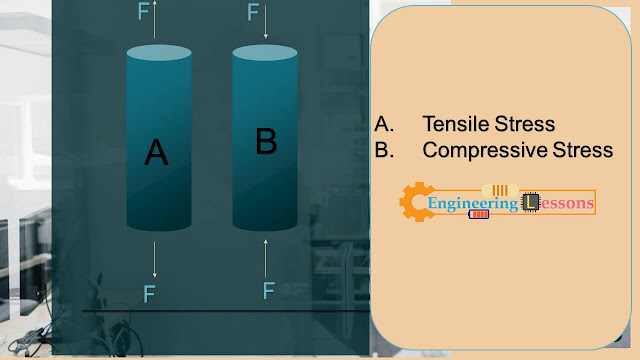# Stress and Strain: An Introduction0

An elastic body can return to its own shape after deformation. An inelastic body cannot return. Deformation is occurred by giving force. Stress and Strain have a relation with deformation and force.

Stress is the cause of deformation and strain is the result of that deformation. For example, “F” unit force is given in spring of “L” unit length as tensile force, the elastic spring will increase in length. Suppose, the new length of the spring is L’. So, the increased length is (L’ – L). This part can be called displacement. And suppose we denote the displacement as “X” unit.

So, here the “F” unit force is the cause of deformation, therefore it is stress. And the “X” unit increment in length is the result of that same deformation; thereby, it is strain.

## Types of Stress

There are mainly two types of stresses.

1. Tensile Stress
2. Compressive Stress

## Tensile Stress:

Tensile stress occurs when two equal and opposite forces are directed away from each other.

## Compressive Stress:

Compressive stress occurs when equal and opposite forces are directed forward to each other.Visual Representation of Tensile and Compressive Stress

### Normal equations to find out stress and strain

Stress = F/A = Force/Area

Strain = (Change of length or Change of Volume or d) / (Initial length or Initial volume or l)

Strain = Change in Length or Volume or angel per unit.

Unit of Stress is Pa, N/m^2, lb/in^2

And as normal, strain does not have any unit.

Now solve an example on stress and strain (Longitudinal Stress and Strain)

Problem: Suppose, there is a steel wire that is 10 m long and 2 mm in diameter is attached to the ceiling and a 300 N weight is attached to the end. What is the applied stress?  Again, if a 20 m steel wire stretches 3.08 mm due to the 300 N load, what is the longitudinal strain?

Solve:

Given that,

Diameter, D = 2 mm = 0.002 m

Force, F = 300 N

Length, L = 10 m

So, Area = 3.1416 x (D/4)^2

= 3.1416 x (0.002/2)^2

= 3.1416 x 10^-6 m^2

Therefore, stress = F/A = 300/3.1416 x 10^-6 = 95492742.55

Now, coming to the later question, we see that given length (L) is 10 m and change in length is 3.08 mm = 0.00308 m.

So the strain = (Change in length)/(Initial Length)

= 0.00308/10 m

= 3.08 x 10^-4

Tags